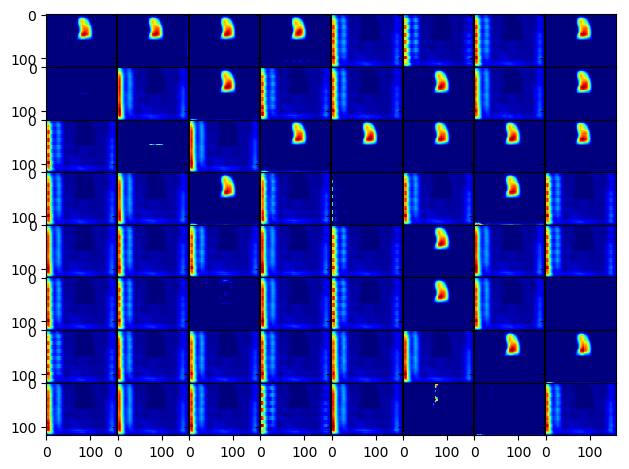# How visualise feature map in original size of input?

(Neda) #1

This snippet visualise the feature map after up2 layer (model was UNet).
First question is how can I display this in the original size of input image. (mapping output of activation in original size).
Second question is how can I get average of all activation and display one image with the original size of input image.

``````criterion = nn.NLLLoss()
optimizer = optim.Adam(model.parameters(), lr=0.00001)  # 1e-3

def visulization():

epoch = 100
PATH = 'D:/Neda/Pytorch/U-net/plots_U_Net/UNet_exp3_epoch/UNet_exp3_epoch{}.pth'.format(epoch)
epoch = checkpoint['epoch']
loss = checkpoint['loss']

model.eval()

activations = {}
test = {}

def get_activation(name):
def hook(model, input, output):
activations[name] = output.detach()
test = copy.deepcopy(activations)
return hook

im_paths = []
target_paths = []
count = 0

for i, data in enumerate(test_loader, 0):
t_image, mask, im_path, target_path = data
im_paths.append(im_path)
target_paths.append(target_path)

flattened_im_paths = [item for sublist in im_paths for item in sublist]
flattened_target_paths = [item for sublist in target_paths for item in sublist]
count +=1

print(count, os.path.basename(flattened_target_paths[i]))

model.up2.register_forward_hook(get_activation('up2')) #torch.Size([1, 128, 120, 160])

outputs = model(t_image)

imgs = activations['up2'].squeeze().split(1, 0) # squeeze the batch dimension and use torch.split to get each channel separately

``````

For example it produced for me this output:each of this channel has size of [120, 160] but how can I display it in original size of image which is [240, 320] and how can I display one image which is average of all channel? (this layer had 128 channels however the above image displays 64 channels)

any comment would be appreciated.

Visualize feature map
(Juan F Montesinos) #2

Cannot answer about an average map. You can use `torch.nn.functional.` `interpolate` and bilinear modeto upsample images (per channel) up to original size.

It works quite well, I upsampled 8x8 to 224x224.

(Neda) #3

@JuanFMontesinos How should I specify the size for interpolate? For example, the output of up2 layer is `#torch.Size([1, 128, 120, 160])` so, it does have 128 channels, and batch is one. the question is what is should be size of input for interpolate to reach to the input original size which was [240, 320]?

``````#torch.Size([1, 128, 120, 160])
imgs = activations['up2'].squeeze().split(1, 0)

imgs = torch.nn.functional.interpolate(imgs, size=None, mode='bilinear')
``````

(Juan F Montesinos) #4

Hi,
I think you have to specify the HxW size like:

``````imgs = torch.nn.functional.interpolate(imgs, size=(240,320), mode='bilinear')
``````

It should deal with channels and batches but I’m not 100 % sure as I have no torch on this device. It deals with batches (at least) for sure.

(Neda) #5

Thank you @JuanFMontesinos . it is causing an error

`if input.dim() == 3 and mode == 'nearest': AttributeError: 'tuple' object has no attribute 'dim'`

if I remove the `torch.split` like `imgs = activations['up2'].squeeze()` then, the size of img is `torch.Size([128, 120, 160])` but it’s causing an error that `Got 3D input, but bilinear mode needs 4D input`

(Juan F Montesinos) #6

Hi,
I’m running it and it works for me.

``````In : o=it(torch.rand(1, 128, 120, 160),size=(240,320))

In : o.size()
Out: torch.Size([1, 128, 240, 320]
``````

it calls

``````torch.nn.functional.interpolate
``````

Which pytorch version are you using? (0.4.1 here)
I don’t know if they modified it in pytorch 1
I think you are putting size in first place, is it possible? the ordering is
interpolate(imgs,size=(240,320))

(Neda) #7

@JuanFMontesinos yes, you are right. thanks a lot. It fixed like this:

``````imgs = activations['up2']
print(imgs.size()) #torch.Size([1, 128, 120, 160])
imgs = torch.nn.functional.interpolate(imgs, size=(240, 320), mode='bilinear')
print(imgs.size()) #torch.Size([1, 128, 240, 320])
``````

how can I display the image after interpolation?

``````fig, axarr = plt.subplots(imgs.size(0))
for idx in range(imgs.size(0)):
axarr[idx].imshow(imgs[idx].cpu().squeeze().numpy(), cmap = 'jet')

plt.show()
save_results_to = 'D:/Neda/Pytorch/U-net/plots_U_Net/UNet_exp3_epoch/visulize_layers_epoch_100/new_plots/test_'
plt.savefig(save_results_to  + str(os.path.basename(flattened_im_paths[i])))
plt.close("all")
``````

not it doesn’t let to plot. `TypeError: 'AxesSubplot' object does not support indexing` how can I plot all of the 128 channels?

(Juan F Montesinos) #8

Hi, you can you `from torchvision.utils import make_grid`
make_grid transforms a batch into a single image by concatenating them.
(Remember to convert the output into a numpy array)